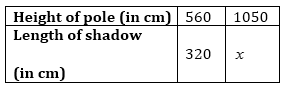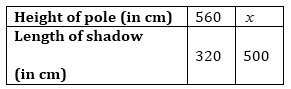# A 5 m 60 cm high vertical pole casts a shadow 3 m 20 cm long. Find at the same time (i) the length of the shadow cast by another pole 10 m 50 cm high (ii) the height of a pole which casts a shadow 5 m long.

Solution

The height of the pole and length of the shadow are in direct proportion.

We know that

1 m = 100 cm

5 m 60 cm = 5×100+60 = 560 cm

3 m 20 cm = 3×100+20 = 320 cm

10 m 50 cm = 10×100+50 = 1050 cm

5 m = 5×100 = 500 cm

(i) Let the length of the shadow of another pole be x.So, 560/320 = 1050/x

x= 1050 x 320 / 560

x= 600 cm = 6m

The length of the shadow of another pole is 6 m.

(ii) Let the height of the pole be x.So, 560/320 = x/500

x= 560 x 500/ 320

x = 8.75 m or 8 m 75 cm

The height of the pole is 8 m 75 cm.